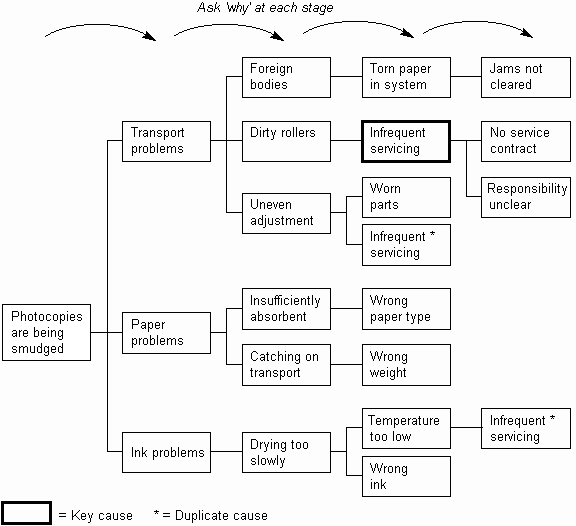The Psychology of Quality and More

# Why-Why Diagram

A Why-Why Diagram is a Tree Diagram where each child statement is determined simply by asking 'why' the parent occurs, as below. It is thus very similar in use to a Cause-Effect Diagram, and techniques may be borrowed from Cause-Effect Diagram usage. Its simplicity can make it useful in less formal situations.

The Why-Why and How-How Diagrams together make a very simple toolset for finding causes of and solutions to problems.How-How Diagram, Tree Diagram, Cause-Effect Diagram

### You can buy books here

And the big
paperback book